# Lesson#59: If A=1, Z=26 then calculate the value of your name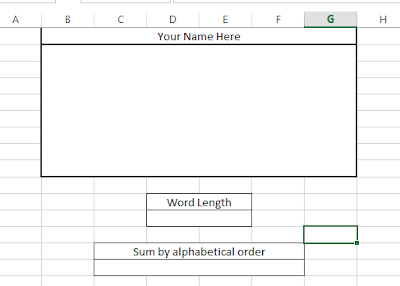This sheet can calculate the value of your name or any word by summing every letter with alphabetical order.

Here I have created a box to input a word.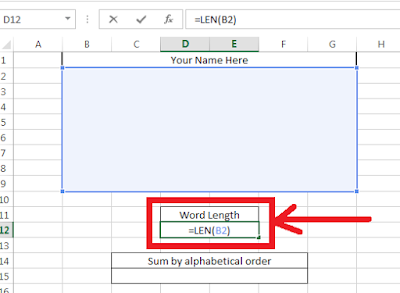I have put this formula =LEN(B2) to calculate the length of the word.Now I have written A-Z in a column and in the next column I have written the order of alphabet.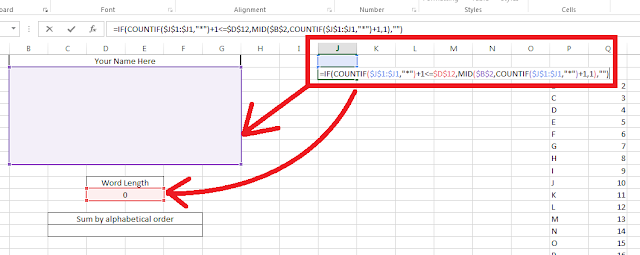Now in a column I have put the formula =IF(COUNTIF(\$J\$1:\$J1,”*”)+1<=\$D\$12,MID(\$B\$2,COUNTIF(\$J\$1:\$J1,”*”)+1,1),””) and I will drag it down in the column. This formula will extract the letter from the word.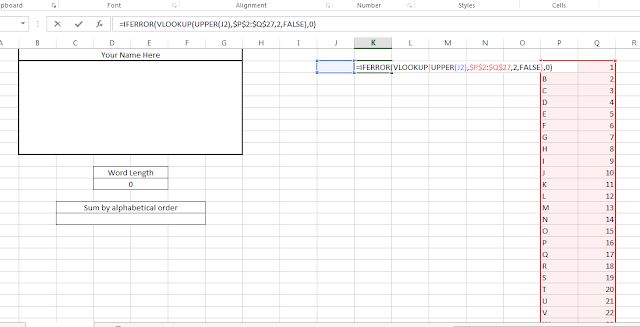In the next column I will put the formula =IFERROR(VLOOKUP(UPPER(J2),\$P\$2:\$Q\$27,2,FALSE),0). This formula will lookup the value of every letter as per alphabetical order.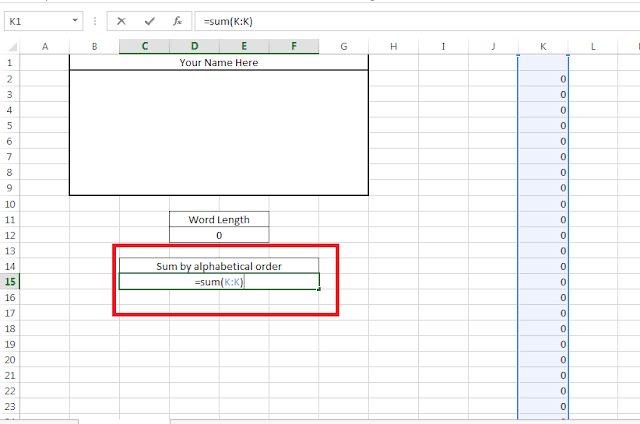Now I have made sum of values.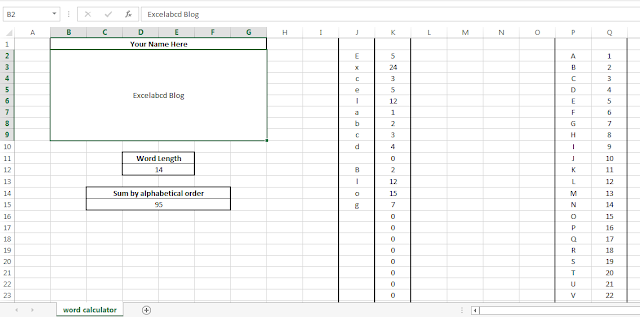This is how it works.